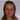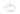# R code and reproducible model development with DVC

In this document we will briefly explore possibilities of a new open source tool that could help with achieving code simplicity, readability and faster model development. There are a lot of example on how to use Data Version Control (DVC) with a Python project. In this document I would like to see how it can be used with a project in R.• Marija Ilić
• July 24, 201716 min readDAG on R example

DVC or Data Version Control tool — its idea is to track files/data dependencies during model development in order to facilitate reproducibility and track data files versioning. Most of the DVC tutorials provide good examples of using DVC with Python language. However, I realized that DVC is a language agnostic tool and can be used with any programming language. In this blog post, we will see how to use DVC in R projects.

## R coding — keep it simple and readable

Each development is always a combination of following steps presented below:

Because of the specificity of the process — iterative development, it is very important to improve some coding and organizational skills. For example, instead of having one big R file with code it is better to split code in several logical files — each responsible for one small piece of work. It is smart to track history development with git tool. Writing “reusable code” is nice skill to have. Put comments in a code can make our life easier.

Beside git, next step in further improvements is to try out and work with DVC. Every time when a change/commit in some of the codes and data sets is made, DVC will reproduce new results with just one bash command on a linux (or Win environment). It memorizes dependencies among files and codes so it can easily repeat all necessary steps/codes instead of us worrying about the order.

## R example — data and code clarification

We’ll take an Python example from DVC tutorial (written by Dmitry Petrov) and rewrite that code in R. With an example we’ll show how can DVC help during development and what are its possibilities.

Firstly, let’s initialize git and dvc on mentioned example and run our codes for the first time. After that we will simulate some changes in the codes and see how DVC works on reproducibility.

R codes can be downloaded from the Github repository. A brief explanation of the codes is presented below:

parsingxml.R — it takes xml that we downloaded from the web and creates appropriate csv file.

 #!/usr/bin/Rscript library(XML) args = commandArgs(trailingOnly=TRUE) if (!length(args)==2) { stop("Two arguments must be supplied (input file name ,output file name - csv ext).n", call.=FALSE) } #read XML line by line con <- file(args, "r") lines <- readLines(con, -1) test <- lapply(lines,function(x){return(xmlTreeParse(x,useInternalNodes = TRUE))}) #parsing XML to get variables ID <- as.numeric(sapply(test,function(x){return(xpathSApply(x, "//row",xmlGetAttr, "Id"))})) Tags <- sapply(test,function(x){return(xpathSApply(x, "//row",xmlGetAttr, "Tags"))}) Title <- as.character(sapply(test,function(x){return(xpathSApply(x, "//row",xmlGetAttr, "Title"))})) Body <- as.character(sapply(test,function(x){return(xpathSApply(x, "//row",xmlGetAttr, "Body"))})) text = paste(Title,Body) label = as.numeric(sapply(Tags,function(x){return(grep("python",x))})) label[is.na(label)]=0 #final data frame for export df <- as.data.frame(cbind(ID,label,text),stringsAsFactors = FALSE) df\$ID=as.numeric(df\$ID) df\$label=as.numeric(df\$label) #write to csv write.csv(df, file=args,row.names=FALSE) print("output file created....")
view raw parsingxml.R hosted with ❤ by GitHub

train_test_spliting.R — stratified sampling by target variable (here we are creating test and train data set)

 #!/usr/bin/Rscript library(caret) args = commandArgs(trailingOnly=TRUE) if (!length(args)==5) { stop("Five arguments must be supplied (input file name, splitting ratio related to test data set, seed, train output file name, test output file name).n", call.=FALSE) } set.seed(as.numeric(args)) df <- read.csv(args,stringsAsFactors = FALSE) test.index <- createDataPartition(df\$label, p = as.numeric(args), list = FALSE) train <- df[-test.index,] test <- df[test.index,] write.csv(train, file=args,row.names=FALSE) write.csv(test, file=args,row.names=FALSE) print("train/test files created....")

featurization.R — text mining and tf-idf matrix creation. In this part we are creating predictive variables.

 #!/usr/bin/Rscript library(text2vec) library(MASS) library(Matrix) args = commandArgs(trailingOnly=TRUE) if (!length(args)==4) { stop("Four arguments must be supplied ( train file (csv format) ,test data set (csv format), train output file name and test output file name - txt files ).n", call.=FALSE) } #read input files df_train = read.csv(args,stringsAsFactors = FALSE) df_test = read.csv(args,stringsAsFactors = FALSE) #create vocabulary - words prep_fun = tolower tok_fun = word_tokenizer it_train = itoken(df_train\$text, preprocessor = prep_fun, tokenizer = tok_fun, ids = df_train\$ID, progressbar = FALSE) vocab = create_vocabulary(it_train,stopwords = stop_words) #clean vocabualary - use only 5000 terms pruned_vocab <- prune_vocabulary(vocab, max_number_of_terms=5000) vectorizer = vocab_vectorizer(pruned_vocab) dtm_train = create_dtm(it_train, vectorizer) #create tf-idf for train data set tfidf = TfIdf\$new() dtm_train_tfidf = fit_transform(dtm_train, tfidf) #create test tf-idf - use vocabulary that is build on train it_test = itoken(df_test\$text, preprocessor = prep_fun, tokenizer = tok_fun, ids = df_test\$ID, progressbar = FALSE) dtm_test_tfidf = create_dtm(it_test, vectorizer) %>% transform(tfidf) #add Id as additional column in matrices dtm_train_tfidf<- Matrix(cbind(label=df_train\$label,dtm_train_tfidf),sparse = TRUE) dtm_test_tfidf<- Matrix(cbind(label=df_test\$label,dtm_test_tfidf),sparse = TRUE) # write output - tf-idf matrices writeMM(dtm_train_tfidf,args) writeMM(dtm_test_tfidf,args) print("Two matrices were created - one for train and one for test data set")
view raw featurization.R hosted with ❤ by GitHub

train_model.R — with created variables we are building logistic regression (LASSO).

 #!/usr/bin/Rscript library(Matrix) library(glmnet) # three arguments needs to be provided - train file (.txt, matrix), seed and output name for RData file args = commandArgs(trailingOnly=TRUE) if (!length(args)==3) { stop("Three arguments must be supplied ( train file (.txt, matrix), seed and argument for RData model name).n", call.=FALSE) } #read train data set trainMM = readMM(args) set.seed(as.numeric(args)) #use regular matrix, not sparse trainMM_reg <- as.matrix(trainMM) t1 = Sys.time() print("Started to train the model... ") glmnet_classifier = cv.glmnet(x = trainMM_reg[,2:500], y = trainMM_reg[,1], family = 'binomial', # L1 penalty alpha = 1, # interested in the area under ROC curve type.measure = "auc", # 5-fold cross-validation nfolds = 5, # high value is less accurate, but has faster training thresh = 1e-3, # again lower number of iterations for faster training maxit = 1e3) print("Model generated...") print(difftime(Sys.time(), t1, units = 'sec')) preds = predict(glmnet_classifier, trainMM_reg[,2:500], type = 'response')[,1] print("AUC for the train... ") glmnet:::auc(trainMM_reg[,1], preds) save(glmnet_classifier,file=args)
view raw train_model.R hosted with ❤ by GitHub

evaluate.R — with trained model we are predicting target on test data set. AUC is final output which is used as evaluation metric.

 #!/usr/bin/Rscript library(Matrix) library(glmnet) args = commandArgs(trailingOnly=TRUE) if (!length(args)==3) { stop("Three arguments must be supplied ( file name where model is stored (RDataname), test file (.txt, matrix) and file name for AUC output).n", call.=FALSE) } #read test data set and model load(args) testMM = readMM(args) testMM_reg <- as.matrix(testMM) #predict test data preds = predict(glmnet_classifier, testMM_reg[,2:500] , type = 'response')[, 1] glmnet:::auc(testMM_reg[,1], preds) #write AUC into txt file write.table(file=args,paste('AUC for the test file is : ',glmnet:::auc(testMM_reg[,1], preds)),row.names = FALSE,col.names = FALSE)
view raw evaluate.r hosted with ❤ by GitHub

Firstly, codes from above we will download into the new folder (clone the repository):

``````\$ mkdir R_DVC_GITHUB_CODE
\$ cd R_DVC_GITHUB_CODE

\$ git clone https://github.com/Zoldin/R_AND_DVC``````

## DVC installation and initialization

On the first site it seemed that DVC will not be compatible to work with R because of the fact that DVC is written in Python and as that needs/requires Python packages and pip package manager. Nevertheless, the tool can be used with any programming language, it is language agnostic and as such is excellent for working with R.

Dvc installation:

``````\$ pip3 install dvc
\$ dvc init``````

With code below 5 R scripts with `dvc run` are executed. Each script is started with some arguments — input and output file names and other parameters (seed, splitting ratio etc). It is important to use `dvc run` — with this command R script are entering pipeline (DAG graph).

``````\$ dvc import https://s3-us-west-2.amazonaws.com/dvc-public/data/tutorial/nlp/25K/Posts.xml.zip \
data/

# Extract XML from the archive.
\$ dvc run tar zxf data/Posts.xml.tgz -C data/

# Prepare data.
\$ dvc run Rscript code/parsingxml.R \
data/Posts.xml \
data/Posts.csv

# Split training and testing dataset. Two output files.
# 0.33 is the test dataset splitting ratio.
# 20170426 is a seed for randomization.
\$ dvc run Rscript code/train_test_spliting.R \
data/Posts.csv 0.33 20170426 \
data/train_post.csv \
data/test_post.csv

# Extract features from text data.
# Two TSV inputs and two pickle matrices outputs.
\$ dvc run Rscript code/featurization.R \
data/train_post.csv \
data/test_post.csv \
data/matrix_train.txt \
data/matrix_test.txt

# Train ML model out of the training dataset.
# 20170426 is another seed value.
\$ dvc run Rscript code/train_model.R \
data/matrix_train.txt 20170426 \
data/glmnet.Rdata

# Evaluate the model by the testing dataset.
\$ dvc run Rscript code/evaluate.R \
data/glmnet.Rdata \
data/matrix_test.txt \
data/evaluation.txt

# The result.
\$ cat data/evaluation.txt``````

## Dependency flow graph on R example

Dependency graph is shown on picture below:

DVC memorizes this dependencies and helps us in each moment to reproduce results.

For example, lets say that we are changing our training model — using ridge penalty instead of lasso penalty (changing alpha parameter to `0`). In that case will change/modify `train_model.R` job and if we want to repeat model development with this algorithm we don’t need to repeat all steps from above, only steps marked red on a picture below:

DVC knows based on DAG graph that changed `train_model.R` file will only change following files: `Glmnet.RData` and `Evaluation.txt`. If we want to see our new results we need to execute only `train_model.R` and `evaluate.R job`. It is cool that we don’t have to think all the time what we need to repeat (which steps). `dvc repro` command will do that instead of us. Here is a code example :

``````\$ vi train_model.R
\$ git commit -am "Ridge penalty instead of lasso"
\$ dvc repro data/evaluation.txt

Reproducing run command for data item data/glmnet.Rdata. Args: Rscript code/train_model.R data/matrix_train.txt 20170426 data/glmnet.Rdata
Reproducing run command for data item data/evaluation.txt. Args: Rscript code/evaluate.R data/glmnet.Rdata data/matrix_test.txt data/evaluation.txt

\$ cat data/evaluation.txt
"AUC for the test file is :  0.947697381983095"``````

`dvc repro` always re executes steps which are affected with the latest developer changes. It knows what needs to be reproduced.

DVC can also work in an "multi-user environment” . Pipelines (dependency graphs) are visible to others colleagues if we are working in a team and using git as our version control tool. Data files can be shared if we set up a cloud and with dvc sync we specify which data can be shared and used for other users. In that case other users can see the shared data and reproduce results with those data and their code changes.

## Summary

DVC tool improves and accelerates iterative development and helps to keep track of ML processes and file dependencies in the simple form. On the R example we saw how DVC memorizes dependency graph and based on that graph re executes only jobs that are related to the latest changes. It can also work in multi-user environment where dependency graphs, codes and data can be shared among multiple users. Because it is language agnostic, DVC allows us to work with multiple programming languages within a single data science project.Subscribe for updates. We won't spam you.## Other Tools

By iterative.ai - an open platform to operationalize AI An open platform to operationalize AI